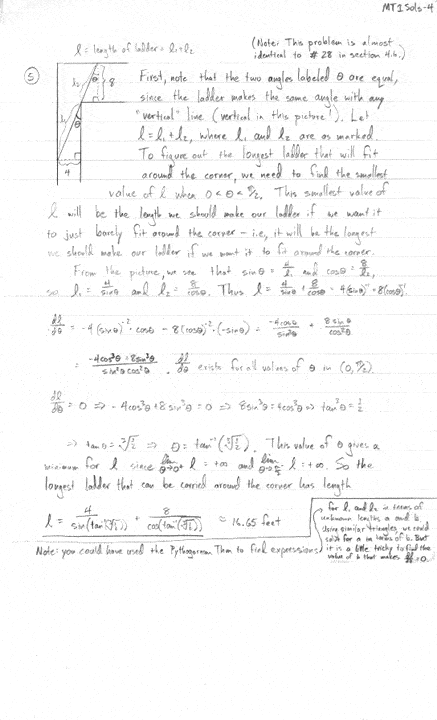# Optimization Hallway Problem

M

#### muna580

##### Guest
I have this optimization problem, with the solution, but I don't really understand how to do this. Can someone please explain it to me? I mean, I the solution, I got totally lost when he started working out the problem after that long paragraph. Where did he get the first equation from?

One hallway (which is 4 feet wide) meets another hallway (which is 8 feet wide) in a right-angled corner. What is the length of the longest ladder which can be carried horizontally around the corner? Give an exact answer, assuming the ladder has no width.#### HallsofIvy

I don't understand what more you want. Do you see why $sin(\theta)= \frac{4}{l_1}$? Do you see why $cos(\theta)= \frac{8}{l_2}$? Do you see why $l_1+ l_2= l$?

### The Physics Forums Way

We Value Quality
• Topics based on mainstream science
• Proper English grammar and spelling
We Value Civility
• Positive and compassionate attitudes
• Patience while debating
We Value Productivity
• Disciplined to remain on-topic
• Recognition of own weaknesses
• Solo and co-op problem solving